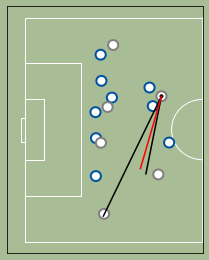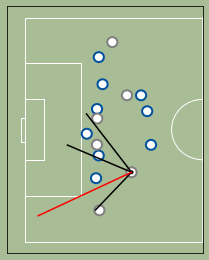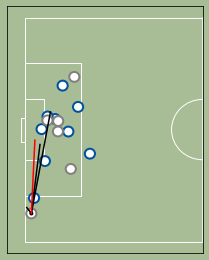### Importing the necessary modules¶

We're using Matplotlib to plot each frame and moviepy to handle the animation. Matplotlib has animation capabilities, but I find moviepy much more simple and clear to use.

In :
import pandas as pd
import numpy as np
from matplotlib import pyplot as plt
from matplotlib.patches import Ellipse
from moviepy.editor import VideoClip
from moviepy.video.io.bindings import mplfig_to_npimage

%matplotlib inline


We are using James' goal for Real versus Barcelona in April 2017. It is a great example of off the ball movement being important in football - an aspect which is mostly ignored by traditional stats, which focus on events, not positioning.

I collected the data myself from watchng the video replay again and again. Don't assume professional level accuracy. This is just for educational/entertainment purposes.

In :
df = pd.read_csv('../datasets/james-vs-barcelona-positonal-data.csv', index_col=(0,1))

colors = {'attack': 'gray',
'defense': '#00529F'}

fps = 20
length = 10


### Drawing the football field¶

In :
X_SIZE = 105.0
Y_SIZE = 68.0

BOX_HEIGHT = (16.5*2 + 7.32)/Y_SIZE*100
BOX_WIDTH = 16.5/X_SIZE*100

GOAL = 7.32/Y_SIZE*100

GOAL_AREA_HEIGHT = 5.4864*2/Y_SIZE*100 + GOAL
GOAL_AREA_WIDTH = 5.4864/X_SIZE*100

def draw_pitch():
"""Sets up field
Returns matplotlib fig and axes objects.
"""

fig = plt.figure(figsize=(X_SIZE/15, Y_SIZE/15))
fig.patch.set_facecolor('#a8bc95')

axes = fig.add_subplot(1, 1, 1, facecolor='#a8bc95')

axes.xaxis.set_visible(False)
axes.yaxis.set_visible(False)

axes.set_xlim(0,100)
axes.set_ylim(0,100)

axes = draw_patches(axes)

return fig, axes

def draw_patches(axes):
plt.xlim([-5,105])
plt.ylim([-5,105])

#pitch
edgecolor="white", facecolor="none", alpha=1))

#half-way line
c='w'))

#penalty areas
ec='w', fc='none'))
ec='w', fc='none'))

#goal areas
ec='w', fc='none'))
ec='w', fc='none'))

#goals
ec='w', fc='none'))
ec='w', fc='none'))

#halfway circle
ec='w', fc='none'))

return axes

draw_pitch()

Out:
(<Figure size 504x326.4 with 1 Axes>,
<matplotlib.axes._subplots.AxesSubplot at 0x1d46e6cd508>)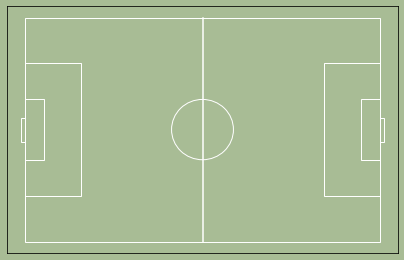### Basic Animation¶

This code will output the basic animation, with the pitch, players and ball plotted. Each frame will then be enhanced with additional metrics in the next versions.

In :
attackers = dfPlayers[dfPlayers.team=='attack'].index
defenders = dfPlayers[dfPlayers.team=='defense'].index

def draw_frame(t, display_num=False):
f = int(t*fps)

fig, ax = draw_pitch()

dfFrame = df.loc[f]

for pid in dfFrame.index:
if pid==0:
size = 0.6
color='black'
edge='black'
else:
size = 3
color='white'
if dfPlayers.loc[pid]['team'] == 'defense':
edge=colors['defense']
else:
edge=colors['attack']

dfFrame.loc[pid]['y']),
size/X_SIZE*100, size/Y_SIZE*100,
edgecolor=edge,
linewidth=2,
facecolor=color,
alpha=1,
zorder=20))
if display_num:
plt.text(dfFrame.loc[pid]['x']-1,dfFrame.loc[pid]['y']-1.3,str(pid),fontsize=8, color='black', zorder=30)

return fig, ax, dfFrame

anim = VideoClip(lambda x: mplfig_to_npimage(draw_frame(x)), duration=length)

#to save the animation to a file, uncomment the next line
#anim.to_videofile('working with positional data - version 1.mp4', fps=fps)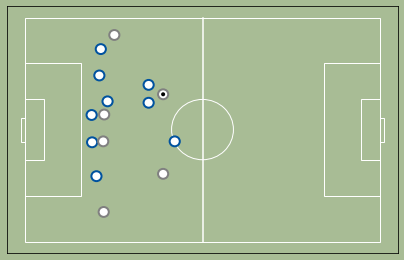## 1. Numerical Superiority¶

As a first approach, let’s define the active zone as the smallest possible area between the goal line and a parallel line that includes the ball and maximizes the attacking superiority. The superiority metric will be the difference between attackers and defenders in the active zone.

In :
def count_players(dfFrame, pid):
count = dfFrame.join(dfPlayers.team)[dfFrame['x']<=dfFrame.loc[pid]['x']].groupby('team').agg('count').max(axis=1)
try:
num_attack = count['attack']
except KeyError:
num_attack = 0
try:
num_defense = count['defense']
except KeyError:
num_defense = 0
return (num_attack-num_defense)

def draw_area(t):
fig, ax, dfFrame = draw_frame(t)

maxX = dfFrame.loc['x']
superiority = count_players(dfFrame, 0)

dfAttackers = dfFrame[(dfFrame.index.get_level_values(0).isin(attackers)) & (dfFrame['x']>maxX)]

for pid, player in dfAttackers.iterrows():
count = count_players(dfFrame, pid)
if count>superiority:
maxX = dfFrame.loc[pid]['x']
superiority = count

if superiority<0:
color='red'
else:
color='black'

plt.text(-5,110,str(superiority),fontsize=25, color=color)

edgecolor="none", facecolor="yellow", alpha=0.1))

return fig, ax

anim = VideoClip(lambda x: mplfig_to_npimage(draw_area(x)), duration=length)

#to save the animation to a file, uncomment the next line
#anim.to_videofile('working with positional data - version 2.mp4', fps=fps)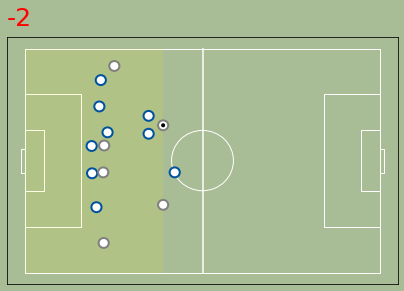## 2. Marking¶

We start by identifying, for each defender, who the closest but farther from the goal attacker is. If the distance to that attacker is less than a certain marking distance (a few meters), we consider that the defender is marking the attacker. If no attacker is closer than that distance, then we assume the defender is marking a zone (a circle with the radius equal to the marking distance).

In :
def closest_player(node, nodes):
nodes = np.asarray(nodes)
deltas = nodes - node
dist = np.einsum('ij,ij->i', deltas, deltas)
return dist.argsort(), dist[dist.argsort()]

def draw_marking(t):
fig, ax, dfFrame = draw_frame(t)

dfAttackers = dfFrame[dfFrame.index.get_level_values(0).isin(attackers)]

for pid in defenders:
circle = False
dfMarking = dfAttackers[dfAttackers['x']>(dfFrame.loc[pid]['x'])]

if dfMarking.shape>0:
closest, closest_dist = closest_player(dfFrame.loc[pid].values,
dfMarking.values)

if closest_dist<75:
[dfFrame.loc[pid]['y'], dfMarking.iloc[closest]['y']],
c='red', zorder=30))
else:
circle = True

else:
circle = True

if circle:
dfFrame.loc[pid]['y']),
10/X_SIZE*100, 10/Y_SIZE*100,
edgecolor='gray',
linewidth=0,
facecolor='gray',
alpha=0.2,
zorder=20))

return fig, ax

anim = VideoClip(lambda x: mplfig_to_npimage(draw_marking(x)), duration=length)

#to save the animation to a file, uncomment the next line
#anim.to_videofile('working with positional data - version 3.mp4', fps=fps)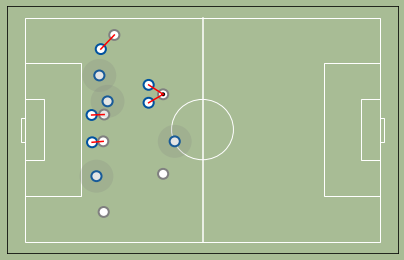## 3. Runs & Passing Options¶

We start by identifying whether each attacker is marked or not. In this case, we are using the expected future attacker position to establish marking — that way, we enable forward runs to open passing options. We also use a different function for the distance (numpy based, so it should be more efficient). Also, for the OCD of you: yes, I know there's a bug in the distance calculation.

We then plot lines between the player who has possession of the ball and all unmarked attackers.

In :
dfFuture = (df.unstack()+df.unstack().diff()*fps).stack()

def draw_passing(t):
fig, ax, dfFrame = draw_frame(t)

if ((dfFrame==dfFrame.loc).sum(axis=1)>1).sum()>1:
f = int(t*fps)
try:
dfFutureFrame = dfFuture.loc[f].join(dfPlayers.team) if len(dfFuture.loc[f])>0 else dfFrame.join(dfPlayers.team)
except:
dfFutureFrame = dfFrame.join(dfPlayers.team)

marked_players = []

for pid in defenders:
dists = dfFutureFrame[(dfFutureFrame.team=='attack') & (dfFutureFrame.x>=dfFrame.loc[pid].x)
].apply(lambda x: np.linalg.norm(x[['x', 'y']]-dfFutureFrame.loc[pid][['x', 'y']]), axis=1)

if len(dists)>0:
if dists.min()<12:
marked_players.append(dists.idxmin())

for pid in attackers:
if pid not in marked_players:
[dfFrame.loc['y'], dfFutureFrame.loc[pid]['y']],
c='black', zorder=30))
return fig, ax

anim = VideoClip(lambda x: mplfig_to_npimage(draw_passing(x)), duration=length)

#to save the animation to a file, uncomment the next line
#anim.to_videofile('working with positional data - version 4.mp4', fps=fps)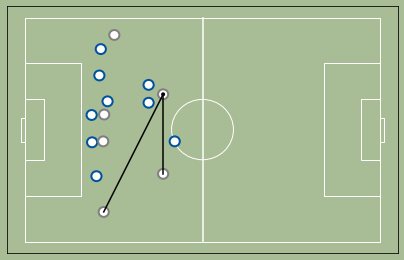## 4. Passing Quality¶

For this metric, we show the actual pass over the passing options (reused from the last metric) at the moment the pass was made.

In :
dfX = df.unstack()['x']

dfChange = df.unstack()[np.sum((dfX.apply(lambda x: x/dfX)==1) !=
(dfX.shift(-1).apply(lambda x: x/dfX.shift(-1))==1)
,axis=1)>0]

for i in range(1,dfChange.shape-2, 2):
f = dfChange.index[i]
f2 = dfChange.index[i+1]
fig, ax = draw_passing(f/fps)